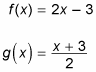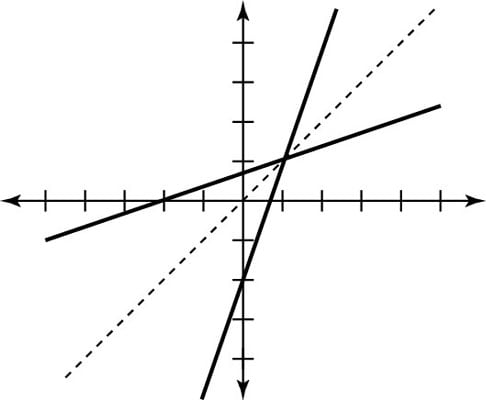##### Pre-Calculus For DummiesIf you’re asked to graph the inverse of a function, you can do so by remembering one fact: a function and its inverse are reflected over the line y = x. This line passes through the origin and has a slope of 1.

When you’re asked to draw a function and its inverse, you may choose to draw this line in as a dotted line; this way, it acts like a big mirror, and you can literally see the points of the function reflecting over the line to become the inverse function points. Reflecting over that line switches the x and the y and gives you a graphical way to find the inverse without plotting tons of points.

The best way to understand this concept is to see it in action. For instance, say that you know these two functions are inverses of each other:To see how x and y switch places, follow these steps:

1. Take a number (any that you want) and plug it into the first given function.

Say you pick –4. When you evaluate f(–4), you get –11. As a point, this is written (–4, –11).

2. Take the value from Step 1 and plug it into the other function.

In this case, you need to find g(–11). When you do, you get –4 back again. As a point, this is (–11, –4). Whoa!

This works with any number and with any function and its inverse: The point (a, b) in the function becomes the point (b, a) in its inverse. But don’t let that terminology fool you. Because they’re still points, you graph them the same way you’ve always been graphing points.

The entire domain and range swap places from a function to its inverse. For instance, knowing that just a few points from the given function f(x) = 2x – 3 include (–4, –11), (–2, –7), and (0, –3), you automatically know that the points on the inverse g(x) will be (–11, –4), (–7, –2), and (–3, 0).

So if you’re asked to graph a function and its inverse, all you have to do is graph the function and then switch all x and y values in each point to graph the inverse. Just look at all those values switching places from the f(x) function to its inverse g(x) (and back again), reflected over the line y = x.You can now graph the function f(x) = 3x – 2 and its inverse without even knowing what its inverse is. Because the given function is a linear function, you can graph it by using the slope-intercept form.

First, graph y = x. The slope-intercept form gives you the y-intercept at (0, –2). Since the slope is 3=3/1, you move up 3 units and over 1 unit to arrive at the point (1, 1). If you move again up 3 units and over 1 unit, you get the point (2, 4). The inverse function, therefore, moves through (–2, 0), (1, 1), and (4, 2). Both the function and its inverse are shown here.